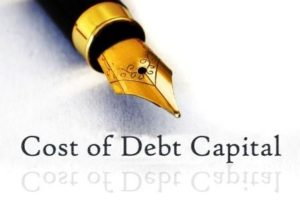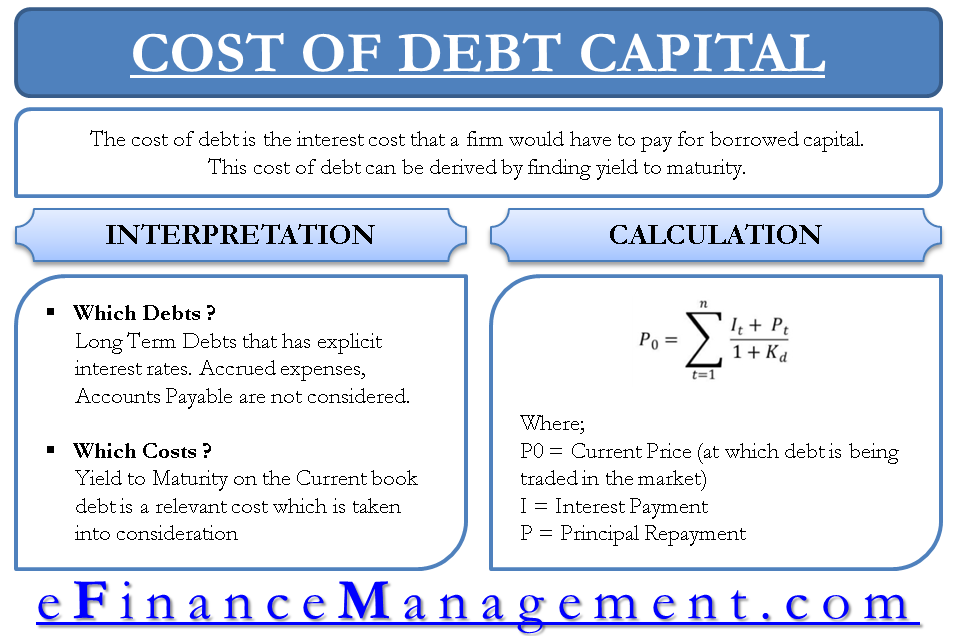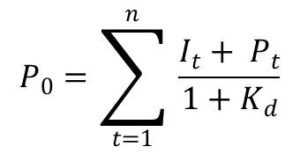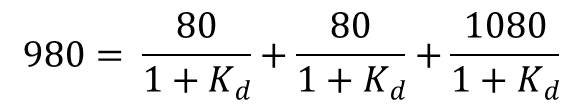# Cost of Debt Capital for Evaluating New Projects – Yield to Maturity

The cost of debt is the interest cost that a firm would have to pay for borrowed capital. Interest cost at which the securities of the firm were issued is the existing cost of capital. This is of no use because for any new project, it is important to see the cost of debt on marginal borrowing by the firm for undertaking the project. This cost of debt can be derived by finding yield to maturity.

Cost of debt capital implies various meaning. We first need to narrow down our focus and be objective. Our objective behind finding the cost of debt is ‘evaluation of new projects’ and using the same in the calculation of weighted average cost of capital. In that light, some obvious questions that stand in front are:

## Which debts are we concerned about and why?

Since our ultimate focus is a financial appraisal of projects, we know that the projects are normally financed by long-term debt. The first filter takes place at this point. We will be concerned with only long-term debt that has explicit interest rates. The accounts payables, accrued expenses etc are not the part of the debt, we are concerned with.

##Which costs are we concerned about and why?

There are can be various understanding of the cost of debt which is as follows:

### Interest cost at which the current book debt was issued.

This cost is not relevant for project evaluation as this is passed and debt at same cost i.e. same interest rate is not necessarily available for current project requirements.

### Current market interest rates.

This again has ambiguity. Interest rate of which kind of security to be considered. The issuers of securities in the market may not have the same kind of risk profile matching the firm we are analyzing. The interest rates or the required return by the borrower would depend on the amount of risk which he is assuming in investing with a particular firm. Hence, the cost of debt may be quite different from the current market interest rates.### Yield to maturity on current book debts.

This is the relevant cost for project evaluation because it represents the cost of debt that will be available for the firm for financing the project. We will see the determination of this cost in the following paragraphs.

## Calculation of Cost of Debt Using Formula / Equation

For a firm, whose debt security is trading at P0 with interest payments ‘I’ and principal repayment ‘P’. The cost of debt is designated by Kd. Kcan be determined by solving the following equation.Explanation of cost of capital with an example

For example, a firm had on the balance sheet, an 8% Debenture which matures after 3 years. The face value is 1000. Putting the formula when the current market price of the debenture is 980, we getSolving the above equation, we will get 8.79%. This is before tax cost of debt capital and therefore, it is not the real cost of capital for us. We still need to give an impact of tax to arrive at the after-tax cost of debt capital. If the tax rate is say 30%, the cost of debt capital is 6.15% {8.79 * (1 – 0.30)}.